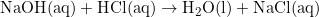×
Get Full Access to Introductory Chemistry - 5 Edition - Chapter 13 - Problem 89p
Get Full Access to Introductory Chemistry - 5 Edition - Chapter 13 - Problem 89p

×

# Solved: Determine the volume of 0.150 M NaOH solution required to neutralize each sampleISBN: 9780321910295 34

## Solution for problem 89P Chapter 13

Introductory Chemistry | 5th Edition

• Textbook Solutions
• 2901 Step-by-step solutions solved by professors and subject experts
• Get 24/7 help from StudySoup virtual teaching assistantsIntroductory Chemistry | 5th Edition

4 5 1 276 Reviews
16
5
Problem 89P

Determine the volume of 0.150 M NaOH solution required to neutralize each sample of hydrochloric acid. The neutralization reaction is:

$$\mathrm {NaOH}(aq)+\mathrm {HCl}(aq) \longrightarrow \mathrm{H_2O}(l)+\mathrm{NaCl}(aq)$$

(a) 25 mL of a 0.150 M HCl solution

(b) 55 mL of a 0.055 M HCl solution

(c) 175 mL of a 0.885 M HCl solution

Equation Transcription:Text Transcription:

NaOH(aq)+HCl(aq) rightarrow H_2O(l)+NaCl(aq)

Step-by-Step Solution:
Step 1 of 8

Step1 of 8

Solution: Here, we are going to calculate the volume of NaOH solution required to neutralize the given HCl solutions.

Neutralisation reaction is the reaction between an acid and a base to give salt and water. The neutralization reaction in the given problem is:Here, 1 mole of HCl requires 1 mole of NaOH for complete neutralization.

___________________

Step 2 of 8

Step 3 of 8

## Discover and learn what students are asking

Calculus: Early Transcendental Functions : The Natural Logarithmic Function: Integration
?In Exercises 1-26, find the indefinite integral. $$\int \frac{9}{5-4 x} d x$$

Statistics: Informed Decisions Using Data : Two-Way Analysis of Variance
?To graphically display the role interaction plays in a factorial design, we draw ______.

Unlock Textbook Solution